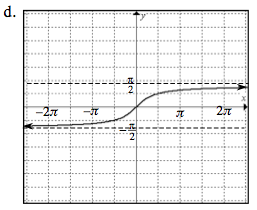### Home > PC > Chapter 6 > Lesson 6.2.1 > Problem6-79

6-79.
1. Find an equation for each graph shown below. Homework Help ✎

1.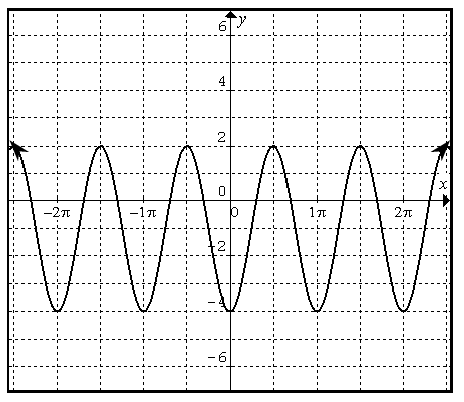2.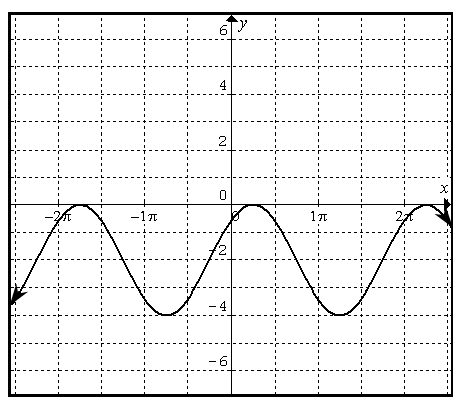3.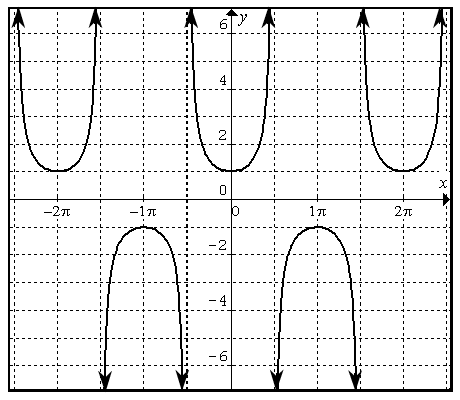4.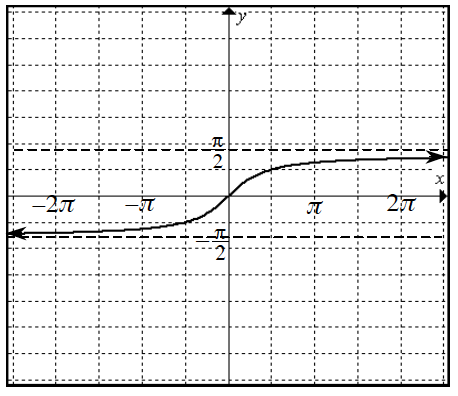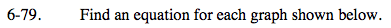Identify the amplitude, period, and any shifting.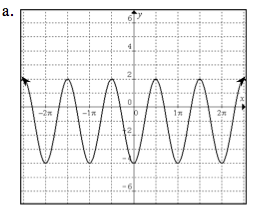Draw max, min, and midlines.

amplitude = 3, period = 1π, shift = down 1Remember that the equation uses 'b' and pb = 2π.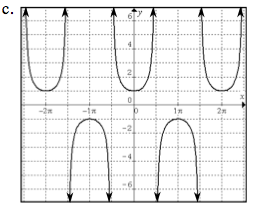These are inverse functions.
Try drawing the non-inverse function.